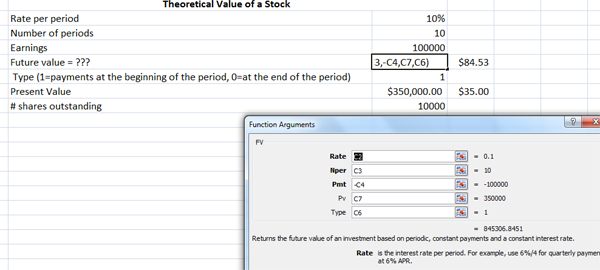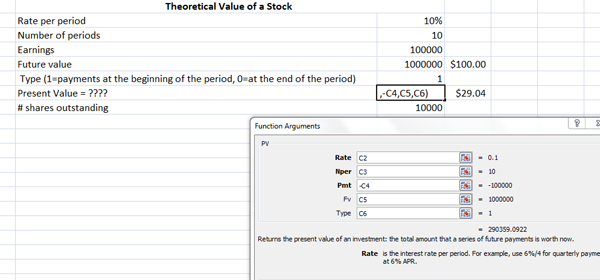# Present and Future Value Using Excel

Post on: 22 Сентябрь, 2016FV(rate,nper,pmt,pv,type)

Rate is the interest rate per period.

Nper is the total number of payment periods in an annuity.

Pmt is the payment made each period; it cannot change over the life of the annuity. Pmt must be entered as a negative number.

Pv is the present value, or the lump-sum amount that a series of future payments is worth right now. If pv is omitted, it is assumed to be 0 (zero). PV must be entered as a negative number.

Type is the number 0 or 1 and indicates when payments are due. If type is omitted, it is assumed to be 0 which represents at the end of the period. If payments are due at the beginning of the period, type should be 1.

PV(rate,nper,pmt,fv,type)

Rate is the interest rate per period. For example, if you obtain an automobile loan at a 10 percent annual interest rate and make monthly payments, your interest rate per month is 10%/12, or 0.83%. You would enter 10%/12, or 0.83%, or 0.0083, into the formula as the rate.

Nper is the total number of payment periods in an annuity. For example, if you get a four-year car loan and make monthly payments, your loan has 4*12 (or 48) periods. You would enter 48 into the formula for nper.

Pmt is the payment made each period and cannot change over the life of the annuity. Pmt must be entered as a negative amount.

Fv is the future value, or a cash balance you want to attain after the last payment is made. Fv must be entered as a negative amount.

Type is the number 0 or 1 and indicates when payments are due. If type is omitted, it is assumed to be 0 which represents at the end of the period. If payments are due at the beginning of the period, type should be 1.

NPV(rate,value1:value29),+cash investment

Rate is the rate of discount over the length of one period.

value1: value29 are 1 to 29 periods representing income.

+cash investment represents the cash investment for the project.

Example:

Example: =NPV(F9,C10:C14),+C9

F9 contains the required rate of return

C10:C14 contains the postive cash flow generated by the project each period

+C9 contains the cash investment required by the project. The cash investment must be entered as a negative amount.

RATE(nper,pmt,pv,fv,type,guess)

Nper is the total number of payment periods in an annuity.

Pmt is the payment made each period and cannot change over the life of the annuity.

Pmt must be entered as a negative amount.

Pv is the present value that the future payment is worth now. Pv must be entered as a negative amount.

Fv is the future value, or a cash balance you want to attain after the last payment is made. If fv is omitted, it is assumed to be 0 (the future value of a loan, for example, is 0).

Type is the number 0 or 1 and indicates when payments are due. If type is omitted, it is assumed to be 0 which represents at the end of the period. If payments are due at the beginning of the period, type should be 1.

Guess is your guess for what the rate will be. If you omit guess, it is assumed to be 10 percent. If RATE does not converge, try different values for guess. RATE usually converges if guess is between 0 and 1.

NPER(rate, pmt, pv, fv, type)

Rate is the interest rate per period.

Pmt is the payment made each period; it cannot change over the life of the annuity. Pmt must be entered as a negative amount.

Pv is the present value, or the lump-sum amount that a series of future payments is worth right now. Pv must be entered as a negative amount.

Fv is the future value, or a cash balance you want to attain after the last payment is made.

Type is the number 0 or 1 and indicates when payments are due. If type is omitted, it is assumed to be 0 which represents at the end of the period. If payments are due at the beginning of the period, type should be 1.

PMT(rate,nper,pv,fv,type)

For a more complete description of the arguments in PMT, see PV.

Rate is the interest rate for the loan.

Nper is the total number of payments for the loan.

Pv is the present value, or the total amount that a series of future payments is worth now; also known as the principal.

Fv is the future value, or a cash balance you want to attain after the last payment is made. If fv is omitted, it is assumed to be 0 (zero), that is, the future value of a loan is 0.Type is the number 0 or 1 and indicates when payments are due. If type is omitted, it is assumed to be 0 which represents at the end of the period. If payments are due at the beginning of the period, type should be 1.

IRR(values,guess)

Values is an array or a reference to cells that contain numbers for which you want to calculate the internal rate of return.

Values must contain at least one positive value and one negative value to calculate the internal rate of return.

IRR uses the order of values to interpret the order of cash flows. Be sure to enter your payment and income values in the sequence you want.

If an array or reference argument contains text, logical values, or empty cells, those values are ignored.

Guess is a number that you guess is close to the result of IRR.

Microsoft Excel uses an iterative technique for calculating IRR. Starting with guess, IRR cycles through the calculation until the result is accurate within 0.00001 percent. If IRR can’t find a result that works after 20 tries, the #NUM! error value is returned.

In most cases you do not need to provide guess for the IRR calculation. If guess is omitted, it is assumed to be 0.1 (10 percent).

If IRR gives the #NUM! error value, or if the result is not close to what you expected, try again with a different value for guess.

Printing Formulas In Excel Worksheets

To display formulas press the Ctrl and

keys at the same time. The

key is generally located at the top left of the keyboard.

On the Excel menu, go to File, Page Setup and click on the Sheet tab as shown below to check the Gridlines and Row and column headings boxes.

Then click the Print Preview button. If the width of the worksheet is greater than the length of the worksheet, click the Setup button and change the orientation of the worksheet to Landscape. If the length of the worksheet is greater than the width use the Portrait oreintation.

Click OK to close the Page Setup screen and then Print the worksheet.

To return the worksheet to normal view, press the Ctrl and

More from this section
Categories
Tags

• Most Popular